https://doi.org/10.5194/angeo-37-549-2019
https://doi.org/10.5194/angeo-37-549-2019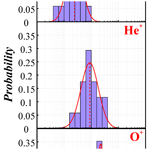# Acceleration of protons and heavy ions to suprathermal energies during dipolarizations in the near-Earth magnetotail

Andrei Y. Malykhin, Elena E. Grigorenko, Elena A. Kronberg, Patrick W. Daly, and Ludmila V. Kozak
Abstract

In this work we present an analysis of the dynamics of suprathermal ions of different masses (H+, He+, O+) during prolonged dipolarizations in the near-Earth magnetotail ($X>-\mathrm{17}{R}_{\mathrm{E}}\right)$ according to Cluster/RAPID observations in 2001–2005. All dipolarizations from our database were associated with fast flow braking and consisted of multiple dipolarization fronts (DFs). We found statistically that fluxes of suprathermal ions started to increase ∼1 min before the dipolarization onset and continued to grow for ∼1 min after the onset. The start of flux growth coincided with the beginning of a decrease in the spectral index γ. The decrease in γ was observed for protons for ∼1 min after the dipolarization onset, and for He+ and O+ ions for ∼3 and ∼5 min after the onset respectively. The negative variations of γ for O+ ions were ∼2.5 times larger than for light ions. This demonstrates more efficient acceleration for heavy ions. The strong negative variations of γ were observed in finite energy ranges for all ion components. This indicates the possibility of nonadiabatic resonant acceleration of ions in the course of their interaction with multiple DFs during dipolarizations. Our analysis showed that some fraction of light ions can be accelerated up to energies ≥600 keV and some fraction of oxygen ions can be accelerated up to ∼1.2 MeV. Such strong energy gains cannot be explained by acceleration at a single propagating DF and suggest the possibility of multistage ion acceleration in the course of their interaction with multiple DFs during the prolonged dipolarizations.

Share
Dates
1 Introduction

One of the important processes in the dynamics of the Earth's magnetotail is magnetic dipolarization. This manifests itself in enhancement of the northward magnetic field component (BZ), which results in transformation of the initially stretched magnetic configuration into the more dipole-like one. This process is often associated with an increase in geomagnetic activity (Sergeev et al., 2012, and references therein).

Spacecraft observations have shown that dipolarization phenomena can be divided into two main groups. The first group includes isolated dipolarization fronts (DFs) propagating along with the bursty bulk flows (BBFs) towards the Earth (e.g., Angelopoulos et al., 1992; Nakamura et al., 2002; Runov et al., 2009). The DFs are usually observed for a few minutes or less (e.g., Schmid et al., 2011), and it is believed that they are formed downtail in the course of reconnection (e.g., Sitnov et al., 2009). The second group includes the so-called “secondary” dipolarizations related to the braking of fast flows and magnetic flux pileup in the near-Earth tail (e.g., Nakamura et al., 2009). The origin of “secondary” dipolarizations is still debated. They can be the consequence of a magnetic flux pileup due to the arrival of multiple BBFs (e.g., Liu et al., 2013, 2014) or they can be caused by the development of cross-tail current instability in the near-Earth CS (e.g., Lui, 2011). Usually the secondary dipolarizations are associated with the formation of the substorm current wedge (SCW) and are observed for up to several hours (McPherron et al., 1973; Sergeev et al., 2012).

The prolonged “secondary” dipolarizations have a complicated temporal and spatial structure (e.g., Nakamura et al., 2009; Grigorenko et al., 2016, 2018; Malykhin et al., 2018a). They consist of the prolonged growth of the BZ field (for  tens of minutes) along with multiple short ( 1–2 min) BZ pulses. It was shown that strong enhancements of the dawn–dusk electric field (EY) are often observed along with the BZ pulses (e.g., Runov et al., 2011; Grigorenko et al., 2018).

Thus, it is natural to assume that such magnetic structures affect the dynamics and acceleration of charged particles. The processes of acceleration of charged particles at single DFs have been studied in detail by using both spacecraft observations and kinetic simulations. Fu et al. (2011) using Cluster observations have shown that electrons experience adiabatic acceleration at DFs by betatron and Fermi mechanisms. On the contrary, spacecraft observations and kinetic simulations demonstrated that ions experience nonadiabatic interaction with the DF and can be resonantly accelerated by its electric field (e.g., Delcourt and Sauvaud, 1994; Delcourt, 2002; Zhou et al., 2010; Greco et al., 2014, 2015; Artemyev et al., 2012, 2015; Ukhorskiy et al., 2013). It was shown that proton acceleration strongly depends on the velocity of the DF, and the proton energy gain increases with the front amplitude (${B}_{{Z}_{max}}$) (Greco et al., 2014). Ukhorskiy et al. (2013) reported that under realistic conditions, the maximum energy gain depends on the dawn–dusk extent of the front. Their simulations demonstrated that the trapped protons can be accelerated up to 100 keV at the DF unbounded in the dawn–dusk direction. The energy gain is limited because of the nonadiabatic scattering off the equatorial plane due to high magnetic field curvature. Greco et al. (2015) demonstrated that the energy gained by the most energetic fraction of ions scales approximately as the square root of the mass ratio and that ion energization at DFs strongly depends on the initial particle energy.

During the prolonged “secondary” dipolarizations the increases in fluxes of suprathermal electrons, protons and heavy ions are often observed (Nosé et al., 2000; Apatenkov et al., 2007; Asano et al., 2010; Grigorenko et al., 2017; Malykhin et al., 2018a, b). Malykhin et al. (2018b) studied the dynamics of fluxes and energy spectra of suprathermal electrons during the prolonged dipolarizations and showed that electrons can be accelerated by a betatron mechanism up to ∼90 keV. On the contrary, the behavior of ion energy spectra indicates the nonadiabatic character of ion acceleration in the course of dipolarizations (e.g., Nosé et al., 2000; Grigorenko et al., 2017; Malykhin et al., 2018a). However, ion dynamics and acceleration mechanisms operating in the course of prolonged dipolarizations are still poorly understood.

In this work, we study the dynamics and acceleration of ions of different masses (H+, He+, O+) to suprathermal energies in the multiscale magnetic structure of dipolarizations in the near-Earth magnetotail by using Cluster/RAPID observations (Wilken et al., 2001). The structure of the article is as follows. In Sect. 2, we describe the observational data used and show a typical example of the dynamics and spectra of suprathermal H+, He+ and O+ ions during dipolarization. In Sect. 3, we present the statistical analysis of the fluxes and spectra of these ion components observed in 17 dipolarization events. The results of our study are formulated and discussed in Sect. 4.

2 Observations

To study the dynamics and acceleration of suprathermal ions we used observations provided by the Research with Adaptive Particle Imaging Detectors (RAPID) spectrometers on board four Clusters spacecraft in the energy range of 40–1500 keV for protons and up to 4000 keV for heavier ions (Wilken et al., 2001). The magnetic field observations were taken from the fluxgate magnetometers (FGMs) (spin- and full-resolution (22.4 Hz) modes were used) (Balogh et al., 2001). Ion moments of thermal population were taken from the COmposition DIstribution Function (CODIF) instrument (Rème et al., 2001). CODIF measures proton fluxes in the energy range of 0–40 keV e−1. The electric field data were provided by the Electric Field and Wave (EFW) instrument (Gustafsson et al., 2001). If not specifically mentioned, we use the geocentric solar magnetospheric (GSM) coordinate system everywhere in the paper.

Figure 1 shows a dipolarization event observed by Cluster-1 (C1) spacecraft on 3 October 2004 between 18:56 and 19:03 UT. The observations from the other Cluster satellites are similar and are not shown. At this time Cluster was located at (−15; 6; 2.5) RE and it was inside the plasma sheet (PS) ($|{B}_{X}|<\mathrm{10}$ nT; see Fig. 1d). The dipolarization started around 18:57:49 UT. This moment is indicated in Fig. 1 by the vertical dotted line. An increase in the earthward ion bulk velocity (VX) was observed simultaneously with the sharp increase in the BZ field (Fig. 1d, f). This indicates the arrival of BBF along with the DF. We will call this DF the onset-related DF, since after this front the dipolarization lasted until  19:45 UT and had the complicated magnetic structure (not shown). Simultaneously with the increase in VX, a sharp increase in the dawn–dusk electric field (EY) was observed. The EY field shown in Fig. 1e represents the smoothed full-resolution EY data by the 4 s sliding average. After the onset of dipolarization the oscillations of the VX value and its sign reversal were observed. This indicates the braking and reflection of the fast flow (e.g., Panov et al., 2010). Multiple short BZ pulses were observed during the BZ growth (between 18:57:49 and 18:58:30 UT) as well as at the later time when the BZ field had already reached the large magnitude.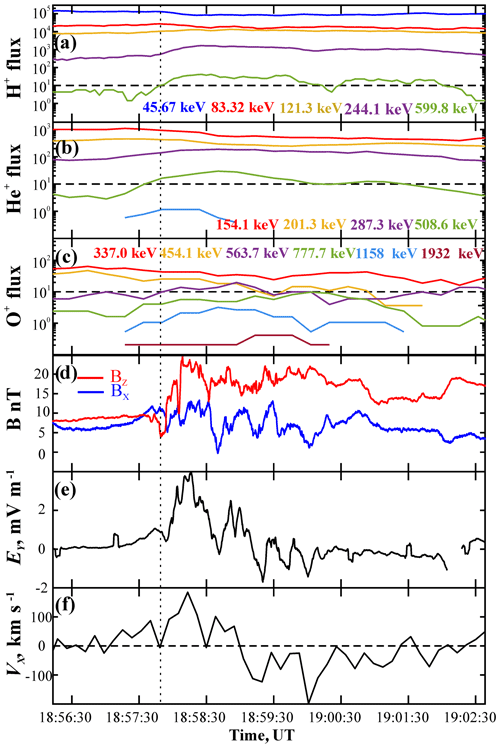Figure 1An overview of the dipolarization event observed on 3 October 2004 by Cluster-1. Panels (a)(c) show the time profiles of fluxes of suprathermal H+, He+ and O+ ions respectively measured by the RAPID instrument in the energy ranges indicated in the corresponding panel. Panel (d) shows time profiles of the BX and BZ components of the magnetic field. Panels (e) and (f) display the dawn–dusk electric field (EY) and X component of ion bulk velocity (VX). The onset of dipolarization is indicated by a vertical dotted line.

Figure 1a–c show the time profiles of suprathermal fluxes of H+, He+ and O+ ions observed in several energy channels of the RAPID instrument. The corresponding average energies are presented in the bottom part of each panel. The increase in fluxes of high-energy ions (∼120–600 keV for H+,  287–1100 keV for He+,  560–1160 keV for O+) started ∼1 min before the dipolarization onset, and the values of these fluxes remained large for several minutes after the onset. At the same time the ion fluxes in a lower energy range ( 45–80 keV for H+,  150–200 keV for He+ and  340–450 keV for O+) decreased during the dipolarization. The contrasting dynamics of ion fluxes in different energy ranges caused the variations in energy spectra and the formation of nonmonotonic features like flattening and bulges.

To quantify the energy spectra of suprathermal ions we used the value of the spectral index γ. To calculate the γ we assume that the ion differential flux (Ji) can be described by a power law at least within the energy range corresponding to neighboring channels of the RAPID instrument: ${J}_{i}\sim {W}^{-\mathit{\gamma }}$, where W is ion kinetic energy (e.g., Øieroset et al., 2002; Imada et al., 2007). We calculated the spectral index γ as it was described by Kronberg and Daly (2013):

$\begin{array}{}\text{(1)}& \mathit{\gamma }=-\mathrm{ln}\left({J}_{i\mathrm{2}}/{J}_{i\mathrm{1}}\right)/\mathrm{ln}\left({E}_{\mathrm{eff}\mathrm{2}}/{E}_{\mathrm{eff}\mathrm{1}}\right).\end{array}$

Here, the Ji2 and Ji1 are the differential fluxes of ions in the neighboring energy channels. The effective energies Eeff2 and Eeff1 were calculated as the geometric mean between the lowest energies of the neighboring channels.

Figure 2 displays the time profiles of γ calculated for three types of ions (H+, He+, O+) for given energy ranges during the interval of interest shown in Fig. 1 (panels b–d). We also calculated the energy spectra of these ion components at the moments shown by the colored vertical lines in Fig. 2a–d and presented them in the right part of the figure. The gray-shaded areas in panels (e)–(g) indicate the energy ranges at which the spectra have nonmonotonic features (flattening and bulges). Figure 2a shows the time profiles of the BZ and BX components for the reference.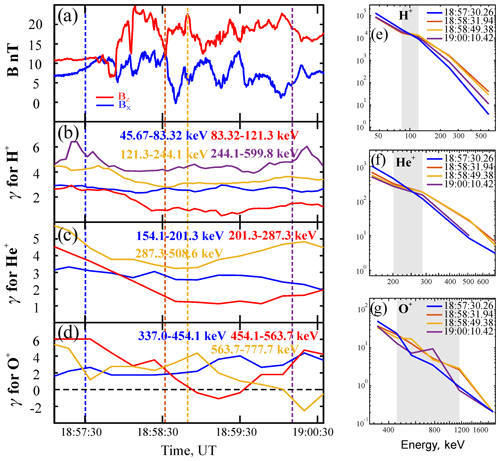Figure 2Variations of spectral index γ calculated for H+ (b), He+ (c) and O+ (d) ion components in the energy ranges indicated in the corresponding panels. Panel (a) shows the time profiles of BX and BZ field for reference. Vertical dashed colored lines indicate the time moments at which energy spectra of H+, He+ and O+ ions are plotted in panels (e)(d) respectively. The gray-shaded areas in panels (e)(d) display the energy ranges at which the peculiarities (flattening or bulges) are observed in the energy spectra.

Before the dipolarization onset the values of the proton's γ in energy ranges  45–83 keV (shown by blue line in Fig. 2b, ${\mathit{\gamma }}_{{\mathrm{H}}_{\mathrm{45}}}$) and ∼83∼121 keV (shown by red line, ${\mathit{\gamma }}_{{\mathrm{H}}_{\mathrm{83}}}$) were similar (∼3.0). After the onset, the ${\mathit{\gamma }}_{{\mathrm{H}}_{\mathrm{45}}}$ almost did not change, but the ${\mathit{\gamma }}_{{\mathrm{H}}_{\mathrm{83}}}$ decreased down to ∼1.5. The decrease in ${\mathit{\gamma }}_{{\mathrm{H}}_{\mathrm{83}}}$ started a few seconds before the onset and lasted until  18:59:20 UT. The corresponding evolution of proton spectra is displayed in Fig. 2e. One can see that the initial spectrum measured at 18:57:30 UT, i.e., before the onset (shown by blue line), has a rather monotonic power-law shape. After the onset the spectrum flattening in the energy range shaded by gray ( 83–121 keV) in Fig. 2e is observed. This feature reflects the observed significant decrease in $|{\mathit{\gamma }}_{{\mathrm{H}}_{\mathrm{83}}}|$. Thus, the proton spectrum becomes more energetic after the dipolarization onset. In the higher energy range ( 121–600 keV) the spectral index γ decreased even earlier (∼1 min before the dipolarization onset) and it proceeded to decrease after the onset. This dynamic was observed simultaneously with the increases in proton fluxes in the corresponding energy channels (see Fig. 1a). The observed negative variations of γ indicate proton acceleration near the onset-related DF as well as within the BZ pulses observed after the onset.

The dynamics of He+ spectra are more or less similar to those of the proton (see Fig. 2c, f). The spectral index γ in the lower energy range  154–201 keV (shown by the blue line in Fig. 2c, ${\mathit{\gamma }}_{{\mathrm{He}}_{\mathrm{154}}}$) decreased slightly for the entire interval of interest. On the contrary, in the higher energy range  201–508 keV the γHe started to decrease ∼1 min before the onset of dipolarization and proceeded to decrease after the onset. The strongest and most prolonged negative variation of γHe was observed in the 201.3–287.3 keV energy range (Fig. 2c). Similarly to protons in the energy spectrum of He+ ions the flattening in a finite energy range ( 201–287 keV) was observed after the dipolarization onset (see the gray-shaded area in Fig. 2f).

The dynamics of energy spectra of oxygen ions are different from the dynamics of light ions spectra. In the lower energy range ( 337–454 keV, shown by blue line in Fig. 2d) the ${\mathit{\gamma }}_{{\mathrm{O}}_{\mathrm{337}}}$ experienced a slight increase over the entire interval of interest. In the higher energy range the fluxes of O+ started to grow ∼30 s before the onset (see Fig. 1c). This manifests in a sharp decrease in ${\mathit{\gamma }}_{{\mathrm{O}}_{\mathrm{563}}}$ (shown by the yellow line in Fig. 2d). Then, after the onset the ${\mathit{\gamma }}_{{\mathrm{O}}_{\mathrm{563}}}$ experienced bipolar variations and its strongest negative variation was observed only by the end of interval of interest, at around 19:00:20 UT. In the middle energy range ( 454–654 keV, shown by red line in Fig. 2d) the decrease in ${\mathit{\gamma }}_{{\mathrm{O}}_{\mathrm{454}}}$ also started before the dipolarization onset, but later than the start of ${\mathit{\gamma }}_{{\mathrm{O}}_{\mathrm{563}}}$ decrease. The decrease in ${\mathit{\gamma }}_{{\mathrm{O}}_{\mathrm{454}}}$ lasted after the dipolarization onset until  18:59:20 UT. Thus, there were time delays between variations of ${\mathit{\gamma }}_{{\mathrm{O}}_{\mathrm{454}}}$ and ${\mathit{\gamma }}_{{\mathrm{O}}_{\mathrm{563}}}$. These features in the dynamics of γ at different energy ranges result in observations of bulges in the energy spectra of O+ ions after the dipolarization onset (see Fig. 2g).

The dynamics of fluxes and energy spectra of different ion components observed before and after the dipolarization onset indicates the ion acceleration in the limited energy ranges, which occurred at different stages of dipolarization for ions of different masses. In the next section we present the statistical analysis of these phenomena.

3 Statistical studies

In the previous section it was shown that fluxes of H+, He+ and O+ ions with energies ≥121, 201 and 454 keV respectively increased during the dipolarization, while ion fluxes in the lower energy range either decreased or remained unchanged. This behavior caused the negative variations of spectral index γ. To study statistically the dynamics of ion fluxes and the γ we applied the superposed epoch analysis to 17 dipolarization events from the list published by Grigorenko et al. (2016), whereas the O+ fluxes were statistically reliable only in 11 events. The list of events used in our statistical studies is presented in Table 1.

Table 1The list of time moments of the dipolarization onset observed in the events from our database. “+” marks those events, in which the reliable fluxes of H+, He+ and O+ ions were detected by RAPID instrument.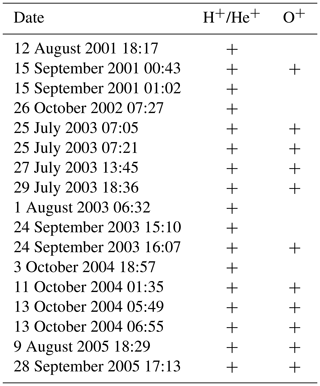Figure 3 shows the epoch profiles of fluxes (panels a–f) and γ (panels g–k) of H+ ions for 17 events listed in Table 1. Figure 3f and k display the epoch profiles of the BZ field. For each event the BZ field was normalized to the maximum value of the BZ observed in a given event: ${B}_{Z}^{\ast }\left(t\right)={B}_{Z}\left(t\right)/{B}_{Zmax}$. The proton fluxes observed in a given energy range were also normalized in a similar way. As the epoch time (t=0) we use the dipolarization onset detected in each event. The black lines display the median profiles of fluxes and ${B}_{Z}^{\ast }\left(t\right)$ and gray dashed lines show lower and upper quartiles of the corresponding epoch profiles.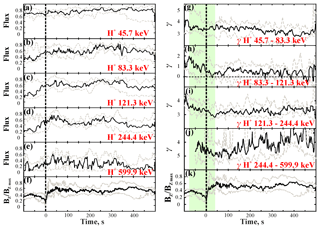Figure 3Panels (a)(e) show the epoch profiles of H+ ion fluxes with energies indicated in the corresponding panel. Panels (g)(j) display the epoch profiles of γ calculated in the given energy ranges indicated in the corresponding panel. Panels (f) and (k) show the epoch profile of the normalized BZ magnetic field (see explanation in the text). The vertical dotted line marks the epoch time t=0, which corresponds to dipolarization onset in each event from our database. The green-shaded area in panels (g)(k) displays the time interval of the γ decrease. The black lines display the median profiles of fluxes and ${B}_{Z}^{\ast }\left(t\right)$ and gray dashed lines show lower and upper quartiles of the corresponding epoch profiles.

The increase in the suprathermal H+ fluxes ( 83–600 keV) started ∼1.5 min before the dipolarization onset and it lasted for ∼1 min after the onset. During this time the γ calculated for this energy range decreased (the green-shaded interval in Fig. 3g–k). However, in the lower energy range (∼45 keV) the proton flux hardly changed (see Fig. 3a), and the corresponding spectral index ${\mathit{\gamma }}_{{\mathrm{H}}_{\mathrm{45}}}$ was almost constant (∼3.5; see Fig. 3g) for all dipolarization intervals. Thus, the decrease in γ was observed only in the limited energy range simultaneously with the flux increase in this range. This indicates that the proton energization reaches energies of ≥83 keV in the course of dipolarizations. It is also worth noting that after the dipolarization onset the ${\mathit{\gamma }}_{{\mathrm{H}}_{\mathrm{83}}}$ decreased down to zero. This indicates the flattening of proton spectra in the finite energy range ( 83–121 keV; see Fig. 3h).

Figure 4 presents the epoch profiles of fluxes (panels a–e) and γ (panels f–i) of He+ ions for 17 events from Table 1. The format of the figure is the same as in Fig. 3. The dynamics of He+ fluxes are similar to the dynamics of H+ fluxes. The monotonic increase in helium fluxes ( 201–508 keV) started ∼1.5 min before the dipolarization onset and lasted for ∼1 min after the onset (see Fig. 4b–d). Conversely the decrease in spectral index γ in  154–508 keV, although it started ∼1 min before the dipolarization onset, was observed for a longer period: for ∼3 min after the onset (see green-shaded interval in Fig. 4f–i). Thus, after the dipolarization onset the acceleration of He+ lasted longer than the proton's acceleration.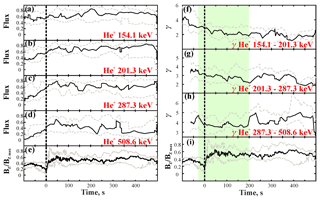Figure 4The epoch profiles for He+ ions. The format of the figure is similar to the format of Fig. 3.

Figure 5 shows the epoch profiles of fluxes (panels a–e) and the γ (panels f–i) of O+ ions observed in 11 dipolarization events from Table 1. The increase in fluxes of O+ ions ( 337–563 keV) was observed at the similar timescale as the increase in energetic H+ and He+ fluxes (see green-shaded interval in Fig. 5a–e). However, the monotonic decrease in γ was observed only in the  454–564 keV energy range. It started just before the dipolarization onset and lasted for ∼5 min after the onset (see green-shaded interval in Fig. 5h). In the lower energy range the γ experienced bipolar variations which started ∼50 s before the onset and were observed for ∼7 min after the onset. The signatures of flattening of energy spectrum (γ∼0) were observed in the energy range of  337–454 keV after the onset (Fig. 5g). It is worth noting that in the energy spectra of oxygen ions the negative variations of γ were much stronger ($\mathrm{\Delta }{\mathit{\gamma }}_{{\mathrm{O}}^{+}}$−5 to −3) than the ones detected in the spectra of light ions ($\mathrm{\Delta }{\mathit{\gamma }}_{{\mathrm{H}}^{+}}$−2, $\mathrm{\Delta }{\mathit{\gamma }}_{{\mathrm{He}}^{+}}$−2.5).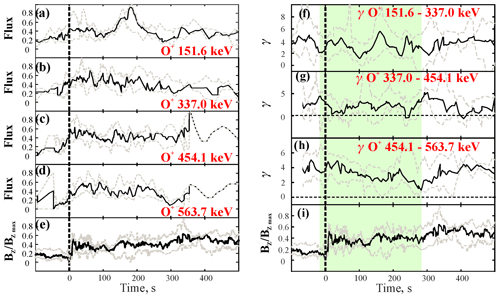Figure 5The epoch profiles for O+ ions. The format of the figure is similar to the format of Fig. 3.

Indeed, in Fig. 6 we present the distribution of probability to observe the given values of Δγ for each ion component and for each energy range calculated for the dipolarization events from our database. The Δγ values were calculated in the following way. For each dipolarization event we determine the maximum value of the spectral index observed around the dipolarization onset (γ0), the minimum value γ1 observed after the onset and the maximum value γ2 observed during the relaxation of γ by the end of the dipolarization. Then, for each event we calculate the negative variation Δγ as γ1γ0, and the positive variation Δγ+ as γ2γ1, and select the strongest one from them. Thus, in Fig. 6 we show the probability to observe a given value of either positive or negative γ variations observed in each dipolarization event. One can see that the $\mathrm{\Delta }{\mathit{\gamma }}_{{\mathrm{H}}^{+}}$ and $\mathrm{\Delta }{\mathit{\gamma }}_{{\mathrm{He}}^{+}}$ values are mainly ranged between −5.0 and 0.0, while the majority of $\mathrm{\Delta }{\mathit{\gamma }}_{{\mathrm{O}}^{+}}$ is ranged between −10.0 and −3.0. Since the negative variations of γ indicate the ion acceleration one may assume that heavy ions (O+) experience stronger acceleration than light ions (H+ and He+) during dipolarizations.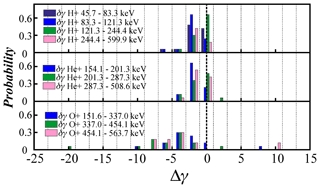Figure 6Distribution of probability to observe the given values of γ variations (see explanation in the text) for H+, He+ and O+ ion components. For each ion component the energy ranges used for γ calculation are indicated by colors according to a legend shown in the corresponding panel.

4 Discussion

In this paper, we analyze the dynamics and spectra of fluxes of different ion components (H+, He+, O+) in suprathermal energy range (45–700 keV) during dipolarizations in the near-Earth magnetotail ($X>-\mathrm{17}{R}_{\mathrm{E}}$) according to Cluster/RAPID observations made in 2001–2005. By using superposed epoch analysis we show that the increase in the suprathermal ion fluxes (H+, He+, O+) started ∼1 min before the onset of dipolarizations, and the fluxes continued to grow for ∼1 min after the onset. The fact that the fluxes of different ion components started to increase simultaneously and the duration of the flux growth is similar for ions of different masses can be related to the contraction of magnetic flux tubes during dipolarization. The contraction of magnetic flux tubes can lead to the increase in ion density, which, in turn, leads to the flux increase. However, our observations demonstrate that the spectral index γ started to decrease almost simultaneously with the flux increase. But, unlike the behavior of ion fluxes, the decrease in γ was observed during different time intervals for different types of ions. Namely, for protons, the decrease in γ was observed for ∼1 min after the onset, while for He+ and O+ ions the γ decreased for ∼3 and ∼5 min after the onset respectively.

The decrease in γ indicates the presence of nonadiabatic acceleration of different ion components during dipolarization. Indeed if the ion acceleration were adiabatic the spectral index should be almost constant (e.g., Pan et al., 2012). However, in all dipolarization events from our database we observed strong negative variations of γ in the limited energy range for all ion components. For O+ ions these variations were in ∼2.5 times larger than for light ions. The amplitude of the negative variations of γ and the duration of the γ decrease demonstrate that oxygen ions experience stronger nonadiabatic acceleration than light ions (H+ and He+). Other important spectral features in favor of a nonadiabatic acceleration mechanism are the flattening of ion spectra and the formation of bulges in a finite energy range.

Theoretical studies and modeling of ion acceleration in a single DF were performed in many papers before (e.g., Ukhorski et al., 2013; Perri et al., 2009; Greco et al., 2010). It was shown that the efficiency of charged-particle acceleration depends not only on the amplitude of the electric field associated with the DF, but also on the spatial structure of this field and the particular location of particle arrival into the acceleration region (Artemyev et al., 2015). Greco et al. (2014) showed that the DF propagating earthward has a complex 2-D electric field structure, which is defined by the front propagation velocity (Vf) and the following spatial scales: magnetic ramp thickness (lf) and DF width (Lf). It was also shown that the acceleration of charged particles also depends on the initial energy and mass of ions (Greco et al., 2015). Thus, heavy ions (O+) should experience more efficient acceleration than light ions. According to their estimations the characteristic energy gain for H+ ions is  20–80 keV, for He+  20–140 keV and for O+  40–240 keV, depending on the DF velocity range, which varies from 200 to 800 km s−1.

Assuming the same acceleration mechanism, we suggest that ions obtain energy by passing the potential drop in the Y direction, in the course of their interaction with the DF. To check this, we estimated the front parameters necessary for such acceleration. By using minimum variance analysis and timing analysis (Sonnerup and Scheible, 1998) we determined the direction and value of the propagation velocity of the DFs in the (XY) plane (Vf) for each dipolarization event from our database (see Table 2). Then, by using the Vf value and the amplitude of the BZ variation (ΔBZ) at the DFs we estimated roughly the electric field associated with the front: $E=\mathrm{\Delta }{B}_{Z}\cdot {V}_{\mathrm{f}}$. Since in the course of nonadiabatic interaction with the DF ions obtain energy at spatial scale of ∼2rL (rL is the ion gyroradius), one can estimate roughly the minimum ion energy gain as $\mathrm{\Delta }W=\mathrm{2}E\cdot {r}_{L}$.

Table 2Parameters of the DFs observed in dipolarization events from our database, namely, the values of the propagation velocity of the DFs (Vf) in the (XY) plane, maximum values of the BZ field (${B}_{{Z}_{max}}$), the amplitude of the BZ field variation (ΔBZ) associated with the DFs, magnetic field value in the dip region observed before the DFs ($|B{|}_{\mathrm{dip}}$) and the maximum values of the magnetic field at the DFs ($|B{|}_{max}$).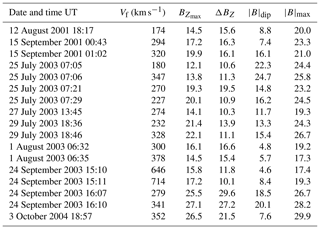It is worth noting that in each dipolarization event from our database we observed the decrease in ion fluxes in the lowest RAPID channels along with the increase in fluxes in higher energy channels. This effect was observed for all types of ions (see Fig. 1). It can be due to the nonadiabatic acceleration of ion populations with initial energies corresponding to the lowest RAPID channels. This results in the replenishment of more energetic ion population and the corresponding flux increase in higher energy channels. Based on these observations, for rL calculation we used the ion energy corresponding to the lowest RAPID channels for each type of ions. Since in the course of ion nonadiabatic interaction with the DF the rL changes both due to the increase in $|B|$ at the front and due to the increase in ion energy, then for the rough estimate of rL we use the average value of the $|B|$: $=\left({B}_{min}+{B}_{max}\right)/\mathrm{2}$, where Bmin is the minimum value of the magnetic field observed at the dip region before the DF (e.g., Shiokawa et al., 2005) and Bmax is the maximum value of the magnetic field corresponding to the DF.

Figure 7 shows the probability distribution of the ΔW of each ion component estimated for all events from our database. The normal mean values for the distributions are ∼24 keV for H+, ∼89 keV for He+ and ∼223 keV for O+ ions. The estimated values of energy gain are enough to transfer some fraction of ion population from the lower part of suprathermal energy distribution to the higher energy range. These results are within the energy ranges obtained by Greco et al. (2015) for the energy gain provided by the nonadiabatic acceleration in the course of ion interaction with a single propagating DF.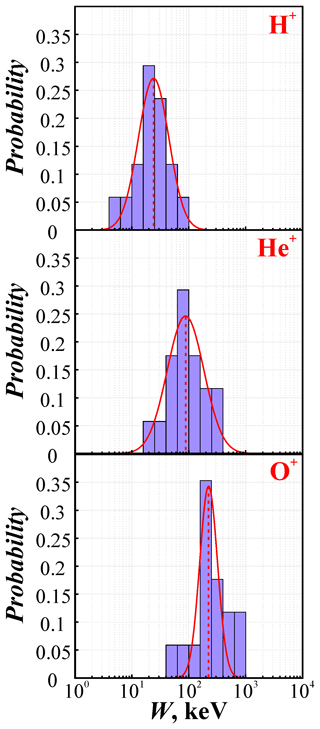Figure 7Histograms of probability to observe the given values of H+, He+ and O+ ion energy gain in the course of nonadiabatic acceleration at the onset-related DF (see explanation in the text).

However, our analysis of the γ dynamics showed that some fraction of light ions can be accelerated to energies of more than 600 keV and some fraction of oxygen ions can be accelerated up to ∼1.2 MeV. Thus, in some cases the amount of energy gain may exceed both the theoretical and our own estimations of ΔW. It is worth noting that these estimations were obtained for a single propagating DF. However, dipolarizations analyzed in our study represent long-lasting complicated events, which consist of multiple DFs with different spatial scales. In the course of interactions with such multiscale magnetic structures ions can experience multistage energy gain. We may suggest that ions are accelerated due to their subsequent nonadiabatic interactions with the system of multiple DFs and, thus, their resulting energy gain can exceed the energy gain estimated for the interaction with a single DF. Verification of this assumption requires simulation of ion dynamics in complicated multiscale dipolarizations.

5 Conclusion

In this paper we studied the dynamics of fluxes and spectra of suprathermal ions of different masses during dipolarizations in the near-Earth geomagnetic tail ($X>-\mathrm{17}{R}_{\mathrm{E}}$) according to Cluster/RAPID observations made in 2001–2005. Below we summarize our main results:

1. During dipolarizations in the near-Earth magnetotail the increase in fluxes of different ion components was observed in the following energy ranges:  120–600 keV for H+,  287–1100 keV for He+ and  560–1160 keV for O+ ions. These increases started ∼1 min before the onset of dipolarizations and lasted for ∼1 min after the onset. Simultaneously with the flux increase in higher energy channels, the decrease in fluxes in lower energies channels was detected. This indicates the presence of nonadiabatic effects in the dynamics of the suprathermal ion fluxes.

2. Simultaneously with the start of flux increase, the decrease in the value of the spectral index γ was observed. The duration and amplitude of negative variations of γ depend on the ion mass. In each dipolarization event from our database the acceleration of heavy ions was observed after the onset during the longer time interval than the acceleration of light ions.

3. The largest amplitude of negative variations in γ was detected for heavy ions (O+). This demonstrates that more efficient acceleration is observed for heavy ions than for light ions (H+, He+).

4. The strong negative variations of γ were observed in finite energy ranges for all ion components. This indicates the possibility of nonadiabatic resonant acceleration of ions in the course of their interaction with multiple DFs during dipolarizations.

Data availability

In this paper we only used open-access data. The Cluster data were downloaded from the Cluster Science Archive version 1.2.1 at http://www.cosmos.esa.int/web/csa (last access: 5 March 2018). To obtain the data, one should start the CSA graphical user interface, and then to download the data, the particular instrument and time interval should be selected.

Author contributions

EEG and AYM wrote the paper and made data analysis. EAK and PWD produced the data and controlled the data quality. LVK calculated theoretical energy gain.

Competing interests

The authors declare that they have no conflict of interest.

Acknowledgements

The work of Andrei Y. Malykhin and Elena E. Grigorenko was supported by the Russian Science Foundation (grant no. 18-47-05001). The work of Elena A. Kronberg and Ludmila V. Kozak was supported by the Volkswagen Foundation (grant Az 90 312). Elena A. Kronberg and Patrick W. Daly acknowledge the “Deutsches Zentrum für Luft und Raumfahrt (DLR)” for the support of the RAPID instrument under grant 50 OC 1602.

Financial support

This research has been supported by the Russian Science Foundation (grant no. 18-47-05001), the Volkswagen Foundation (grant no. Az 90 312), and the Deutsches Zentrum für Luft und Raumfahrt (DLR) (grant no. 50 OC 1602).

Review statement

This paper was edited by Nick Sergis and reviewed by two anonymous referees.

References

Angelopoulos, V., Baumjohann, W., Kennel, C. F., Coroniti, F. V., Kivelson, M. G., Pellat, R., Walker, R. J., Lühr, H., and Paschmann, G.: Bursty bulk flows in the inner central plasma sheet, J. Geophys. Res., 97, 4027–4039, https://doi.org/10.1029/91JA02701, 1992.

Apatenkov, S. V., Sergeev, V. A., Kubyshkina, M. V., Nakamura, R., Baumjohann, W., Runov, A., Alexeev, I., Fazakerley, A., Frey, H., Muhlbachler, S., Daly, P. W., Sauvaud, J.-A., Ganushkina, N., Pulkkinen, T., Reeves, G. D., and Khotyaintsev, Y.: Multi-spacecraft observation of plasma dipolarization/injection in the inner magnetosphere, Ann. Geophys., 25, 801–814, https://doi.org/10.5194/angeo-25-801-2007, 2007.

Artemyev, A. V., Lutsenko, V. N., and Petrukovich, A. A.: Ion resonance acceleration by dipolarization fronts: analytic theory and spacecraft observation, Ann. Geophys., 30, 317–324, https://doi.org/10.5194/angeo-30-317-2012, 2012.

Artemyev, A. V., Liu, J., Angelopoulos, V., and Runov, A.: Acceleration of ions by electric field pulses in the inner magnetosphere, J. Geophys. Res.-Space, 120, 4628–4640, https://doi.org/10.1002/2015JA021160, 2015.

Asano, Y., Shinohara, I., Retinò, A., Daly, P. W., Kronberg, E. A., Takada, T., Nakamura, R., Khotyaintsev, Y. V., Vaivads, A., Nagai, T., Baumjohann, W., Fazakerley, A. N., Owen, C. J., Miyashita, Y., Lucek, E. A., and Rème, H.: Electron acceleration signatures in the magnetotail associated with substorms, J. Geophys. Res., 115, A05215, https://doi.org/10.1029/2009JA014587, 2010.

Balogh, A., Carr, C. M., Acuña, M. H., Dunlop, M. W., Beek, T. J., Brown, P., Fornacon, K.-H., Georgescu, E., Glassmeier, K.-H., Harris, J., Musmann, G., Oddy, T., and Schwingenschuh, K.: The Cluster Magnetic Field Investigation: overview of in-flight performance and initial results, Ann. Geophys., 19, 1207–1217, https://doi.org/10.5194/angeo-19-1207-2001, 2001.

Delcourt, D. C.: Particle acceleration by inductive electric fields in the inner magnetosphere, J. Atmos. Sol.-Terr. Phy., 64, 551–559, 2002.

Delcourt, D. C. and Sauvaud, J. A.: Plasma sheet ion energization during dipolarization events, J. Geophys. Res., 99, 97–108, https://doi.org/10.1029/93JA01895, 1994.

Fu, H. S., Khotyaintsev, Y. V., André, M., and Vaivads, A.: Fermi and betatron acceleration of suprathermal electrons behind dipolarization fronts, Geophys. Res. Lett., 38, L16104, https://doi.org/10.1029/2011GL048528, 2011.

Greco, A., Perri, S., and Zimbardo, G.: Stochastic Fermi acceleration in the magnetotail current sheet: A numerical study, J. Geophys. Res., 115, A02203, https://doi.org/10.1029/2009JA014690, 2010.

Greco, A., Artemyev, A., and Zimbardo, G.: Proton acceleration at two-dimensional dipolarization fronts in the magnetotail, J. Geophys. Res.-Space, 119, 8929–8941, https://doi.org/10.1002/2014JA020421, 2014.

Greco, A., Artemyev, A., and Zimbardo, G.: Heavy ion acceleration at dipolarization fronts in planetary magnetotails, Geophys. Res. Lett., 42, 8280–8287, https://doi.org/10.1002/2015GL066167, 2015.

Grigorenko, E. E., Kronberg, E. A., Daly, P. W., Ganushkina, N. Yu., Lavraud, B., Sauvaud, J.-A., and Zelenyi, L. M.: Origin of low proton-to-electron temperature ratio in the Earth's plasma sheet, J. Geophys. Res.-Space, 121, 9985–10004, https://doi.org/10.1002/2016JA022874, 2016.

Grigorenko, E. E., Kronberg, E. A., and Daly, P. W.: Heating and Acceleration of Charged Particles during Magnetic Dipolarizations, Cosmic Res.+, 55, 57–66, 2017.

Grigorenko, E. E., Dubyagin, S., Malykhin, A. Yu., Khotyaintsev, Yu. V., Kronberg, E. A., Lavraud, B., and Ganushkina, N. Yu.: Intense current structures observed at electron kinetic scales in the near-Earth magnetotail during dipolarization and substorm current wedge formation, Geophys. Res. Lett., 45, 602–611, https://doi.org/10.1002/2017GL076303, 2018.

Gustafsson, G., André, M., Carozzi, T., Eriksson, A. I., Fälthammar, C.-G., Grard, R., Holmgren, G., Holtet, J. A., Ivchenko, N., Karlsson, T., Khotyaintsev, Y., Klimov, S., Laakso, H., Lindqvist, P.-A., Lybekk, B., Marklund, G., Mozer, F., Mursula, K., Pedersen, A., Popielawska, B., Savin, S., Stasiewicz, K., Tanskanen, P., Vaivads, A., and Wahlund, J.-E.: First results of electric field and density observations by Cluster EFW based on initial months of operation, Ann. Geophys., 19, 1219–1240, https://doi.org/10.5194/angeo-19-1219-2001, 2001.

Imada, S., Nakamura, R., Daly, P.W., Hoshino, M., Baumjohann, W., Muhlbachler, S., Balogh, A., and Reme, H.: Energetic electron acceleration in the downstream reconnection outflow region, J. Geophys. Res., 112, A03202, https://doi.org/10.1029/2006JA011847, 2007.

Kronberg, E. A. and Daly, P. W.: Spectral analysis for wide energy channels, Geosci. Instrum. Method. Data Syst., 2, 257–261, https://doi.org/10.5194/gi-2-257-2013, 2013.

Liu, J., Angelopoulos, V., Runov, A., and Zhou, X.-Z.: On the current sheets surrounding dipolarizing flux bundles in the magnetotail. The case of wedgelets, J. Geophys. Res., 118, 2000–2020, https://doi.org/10.1002/jgra.50092, 2013.

Liu, J., Angelopoulos, V., Runov, A., and Zhou, X.-Z.: Magnetic flux transport by dipolarizing flux bundles, J. Geophys. Res., 119, 909–926, https://doi.org/10.1002/2013JA019395, 2014.

Lui, A. T. Y.: Reduction of the cross-tail current during near-Earth dipolarization with multisatellite observations, J. Geophys. Res., 116, A12239, https://doi.org/10.1029/2011JA017107, 2011.

Malykhin, A. Y., Grigorenko, E. E., Kronberg, E. A., Koleva, R., Ganushkina, N. Y., Kozak, L., and Daly, P. W.: Contrasting dynamics of electrons and protons in the near-Earth plasma sheet during dipolarization, Ann. Geophys., 36, 741–760, https://doi.org/10.5194/angeo-36-741-2018, 2018a.

Malykhin, A. Yu., Grigorenko, E. E., Kronberg, E. A., and Daly, P. W.: The Effect of the Betatron Mechanism on the Dynamics of Superthermal Electron Fluxes within Dipolizations in the Magnetotail, Geomagn. Aeronomy+, 58, 744–752, https://doi.org/10.1134/S0016793218060099, 2018b.

McPherron, R. L., Russell, C. T., and Aubry, M. A.: Satellite studies of magnetospheric substorms on august 15, 1968, 9, phenomenological model for substorms, J. Geophys. Res., 78, 3131–3149, https://doi.org/10.1029/JA078i016p03131, 1973.

Nakamura, R., Baumjohann, W., Klecker, B., Bogdanova, Y., Balogh, A., Reme, H., Bosqued, J. M., Dandouras, I., Sauvaud, J. A., Glassmeier, K. H., Kistler, L., Mouikis, C., Zhang, T. L., Eichelberger, H., and Runov, A.: Motion of the dipolarization front during a flow burst event observed by Cluster, Geophys. Res. Lett., 29, 1942, https://doi.org/10.1029/2002GL015763, 2002.

Nakamura, R., Retinò, A., Baumjohann, W., Volwerk, M., Erkaev, N., Klecker, B., Lucek, E. A., Dandouras, I., André, M., and Khotyaintsev, Y.: Evolution of dipolarization in the near-Earth current sheet induced by Earthward rapid flux transport, Ann. Geophys., 27, 1743–1754, https://doi.org/10.5194/angeo-27-1743-2009, 2009.

Nosé, M., Lui, A. T. Y., Ohtani, S., Mauk, B. H., McEntire, R. W., Williams, D. J., Mukai, T., and Yumoto, K.: Acceleration of oxygen ions of ionospheric origin in the near-Earth magnetotail during substorms, J. Geophys. Res., 105, 7669, https://doi.org/10.1029/1999JA000318, 2000.

Øieroset, M., Lin, R. P., Phan, T. D., Larson, D. E., and Bale, S. D.: Evidence for Electron Acceleration up to ∼300 keV in the Magnetic Reconnection Diffusion Region of Earth's Magnetotail, Phys. Rev. Lett., 89, 195001, https://doi.org/10.1029/2012JA018156, 2002.

Pan, Q., Ashour-Abdalla, M., El-Alaoui, M., Walker, R. J., and Goldstein, M. L.: Adiabatic acceleration of suprathermal electrons associated with dipolarization fronts, J. Geophys. Res., 117, A12224, https://doi.org/10.1029/2012JA018156, 2012.

Panov, E. V., Nakamura, R., Baumjohann, W., Angelopoulos, V., Petrukovich, A. A., Retinò, A., Volwerk, M., Takada, T., Glassmeier, K. H., McFadden, J. P., and Larson, D.: Multiple overshoot and rebound of a bursty bulk flow, Geophys. Res. Lett., 37, L08103, https://doi.org/10.1029/2009GL041971, 2010.

Perri, S., Greco, A., and Zimbardo, G.: Stochastic and direct acceleration mechanisms in the Earth's magnetotail, Geophys. Res. Lett., 36, L04103, https://doi.org/10.1029/2008GL036619, 2009.

Rème, H., Aoustin, C., Bosqued, J. M., Dandouras, I., Lavraud, B., Sauvaud, J. A., Barthe, A., Bouyssou, J., Camus, Th., Coeur-Joly, O., Cros, A., Cuvilo, J., Ducay, F., Garbarowitz, Y., Medale, J. L., Penou, E., Perrier, H., Romefort, D., Rouzaud, J., Vallat, C., Alcaydé, D., Jacquey, C., Mazelle, C., d'Uston, C., Möbius, E., Kistler, L. M., Crocker, K., Granoff, M., Mouikis, C., Popecki, M., Vosbury, M., Klecker, B., Hovestadt, D., Kucharek, H., Kuenneth, E., Paschmann, G., Scholer, M., Sckopke, N., Seidenschwang, E., Carlson, C. W., Curtis, D. W., Ingraham, C., Lin, R. P., McFadden, J. P., Parks, G. K., Phan, T., Formisano, V., Amata, E., Bavassano-Cattaneo, M. B., Baldetti, P., Bruno, R., Chionchio, G., Di Lellis, A., Marcucci, M. F., Pallocchia, G., Korth, A., Daly, P. W., Graeve, B., Rosenbauer, H., Vasyliunas, V., McCarthy, M., Wilber, M., Eliasson, L., Lundin, R., Olsen, S., Shelley, E. G., Fuselier, S., Ghielmetti, A. G., Lennartsson, W., Escoubet, C. P., Balsiger, H., Friedel, R., Cao, J.-B., Kovrazhkin, R. A., Papamastorakis, I., Pellat, R., Scudder, J., and Sonnerup, B.: First multispacecraft ion measurements in and near the Earth's magnetosphere with the identical Cluster ion spectrometry (CIS) experiment, Ann. Geophys., 19, 1303–1354, https://doi.org/10.5194/angeo-19-1303-2001, 2001.

Runov, A., Angelopoulos, V., Sitnov, M. I., Sergeev, V. A., Bonnell, J., McFadden, J. P., Larson, D., Glassmeier, K.-H., and Auster, U.: THEMIS observations of an earthward-propagating dipolarization front, Geophys. Res. Lett., 36, L14106, https://doi.org/10.1029/2009GL038980, 2009.

Runov, A., Angelopoulos, V., Zhou, X.-Z., Zhang, X.-J., Li, S., Plaschke, F., and Bonnell, J.: A THEMIS multicase study of dipolarization fronts in the magnetotail plasma sheet, J. Geophys. Res., 116, A052216, https://doi.org/10.1029/2010JA016316, 2011.

Schmid, D., Volwerk, M., Nakamura, R., Baumjohann, W., and Heyn, M.: A statistical and event study of magnetotail dipolarization fronts, Ann. Geophys., 29, 1537–1547, https://doi.org/10.5194/angeo-29-1537-2011, 2011.

Sergeev, V. A., Angelopoulos, V., and Nakamura, R.: Recent advances in understanding substorm dynamics, Geophys. Res. Lett., 39, L05101, https://doi.org/10.1029/2012GL050859, 2012.

Shiokawa, K., Miyashita, Y., Sinohara, I., and Matsuoka, A.: Decrease in BZ prior to the dipolarization in the near-Earth plasma sheet, J. Geophys. Res., 110, A09219, https://doi.org/10.1029/2005JA011144, 2005.

Sitnov, M. I, Swisdak, M., and Divin, A. V.: Dipolarization fronts as a signature of transient reconnection in the magnetotail, J. Geophys. Res., 114, A04202, https://doi.org/10.1029/2008JA013980, 2009.

Sonnerup, B. U. Ö. and Scheible M.: Analysis Methods for Multi-Spacecraft Data, edited by: Pashmann, G. and Daly, P. W., ISSI Scientific Report SR-001, Bern, Chap. 8, p. 185, available at: http://www.issibern.ch/PDF-Files/analysis_methods_1_1a.pdf (last access: 6 July 2019), 1998.

Ukhorskiy, A. Y., Sitnov, M. I., Merkin, V. G., and Artemyev, A. V.: Rapid acceleration of protons upstream of earthward propagating dipolarization fronts, J. Geophys. Res.-Space, 118, 4952–4962, https://doi.org/10.1002/jgra.50452, 2013.

Wilken, B., Daly, P. W., Mall, U., Aarsnes, K., Baker, D. N., Belian, R. D., Blake, J. B., Borg, H., Büchner, J., Carter, M., Fennell, J. F., Friedel, R., Fritz, T. A., Gliem, F., Grande, M., Kecskemety, K., Kettmann, G., Korth, A., Livi, S., McKenna-Lawlor, S., Mursula, K., Nikutowski, B., Perry, C. H., Pu, Z. Y., Roeder, J., Reeves, G. D., Sarris, E. T., Sandahl, I., Søraas, F., Woch, J., and Zong, Q.-G.: First results from the RAPID imaging energetic particle spectrometer on board Cluster, Ann. Geophys., 19, 1355–1366, https://doi.org/10.5194/angeo-19-1355-2001, 2001.

Zhou, X., Angelopoulos, V., Sergeev, V. A., and Runov, A.: Accelerated ions ahead of earthward propagating dipolarization fronts, J. Geophys. Res., 115, A00I03, https://doi.org/10.1029/2010JA015481, 2010.